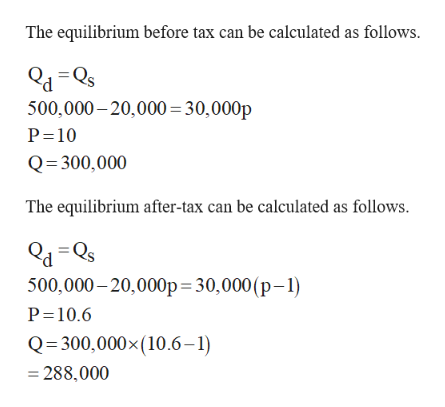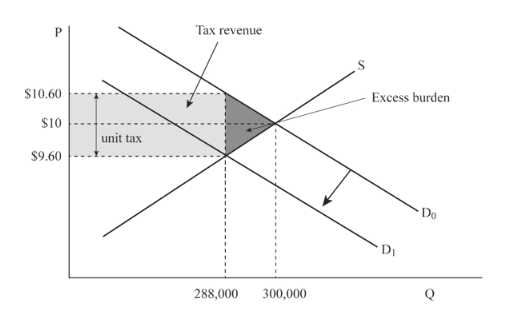# .12) In the effort to reduce alcohol consumption, thegovernment is considering a \$1 tax on each gallon of liquor sold. The legal incidence of the taxwill be on producers. Suppose the demand for alcohol is described by QD= 500,000 – 20,000*Pwhere QDis quantity and P is price per gallon (NOTE: theinversedemand curve would be P = 25– 0.00005*QD). The supply curve is described at QS= 30,000*P (NOTE: This would make the MCcurve MC = (1/30,000)*QS).a.Draw the supply and demand curves before the tax is imposed. Calculate theequilibrium price and quantity.b.Add the tax to the supply curve. Calculate the new price per gallon consumers pay, theprice per gallon producers receive, and the new equilibrium quantity.c.Calculate the amount of revenue the tax generates. How much of the tax is paid byconsumers? How much of the tax is paid by producers?d.Calculate the elasticity of demand at the original equilibrium price. Calculate theelasticity of supply at the original equilibrium price.e.Calculate the deadweight loss of the tax.f.Suppose that if you were to disaggregate the market demand into young drinkers andold drinkers you would find that the demand for alcohol is more elastic among youngdrinkers than old drinkers. Which group of drinkers will change their behavior more?Which group of drinkers will bear the bigger burden of the proportion of the tax thatfalls on consumers? Expla

Question
153 views
.12) In the effort to reduce alcohol consumption, the
government is considering a \$1 tax on each gallon of liquor sold. The legal incidence of the tax
will be on producers. Suppose the demand for alcohol is described by Q
D
= 500,000 – 20,000*P
where Q
D
is quantity and P is price per gallon (NOTE: the
inverse
demand curve would be P = 25
– 0.00005*Q
D
). The supply curve is described at Q
S
= 30,000*P (NOTE: This would make the MC
curve MC = (1/30,000)*Q
S
).
a.
Draw the supply and demand curves before the tax is imposed. Calculate the
equilibrium price and quantity.
b.
Add the tax to the supply curve. Calculate the new price per gallon consumers pay, the
price per gallon producers receive, and the new equilibrium quantity.
c.
Calculate the amount of revenue the tax generates. How much of the tax is paid by
consumers? How much of the tax is paid by producers?
d.
Calculate the elasticity of demand at the original equilibrium price. Calculate the
elasticity of supply at the original equilibrium price.
e.
Calculate the deadweight loss of the tax.
f.
Suppose that if you were to disaggregate the market demand into young drinkers and
old drinkers you would find that the demand for alcohol is more elastic among young
drinkers than old drinkers. Which group of drinkers will change their behavior more?
Which group of drinkers will bear the bigger burden of the proportion of the tax that
falls on consumers? Expla
check_circle

Step 1

Hey, Thank you for the question. According to our policy we can only answer up to 3 subparts per session. If you need further help, post the question again and mention the number that you want us to solve.

Step 2

(a)

The equilibrium before and after tax can be calculated as follows.help_outlineImage TranscriptioncloseThe equilibrium before tax can be calculated as follows. 500,000-20,000 30,000p P 10 Q 300,000 The equilibrium after-tax can be calculated as follows. d=Qs 500,000-20,000p 30,000 (p-1) P 10.6 Q 300,000x (10.6-1) 288,000 fullscreen
Step 3

Consumers pay \$10.5 and producers \$9.6 as \$1 goes for tax. Using th...help_outlineImage TranscriptioncloseTax revenue Excess burden \$10.60 \$10 unit tax \$9.60 Do DI Q 300,000 288,000 fullscreen

### Want to see the full answer?

See Solution

#### Want to see this answer and more?

Solutions are written by subject experts who are available 24/7. Questions are typically answered within 1 hour.*

See Solution
*Response times may vary by subject and question.
Tagged in